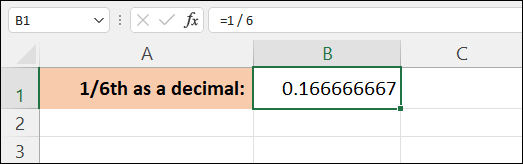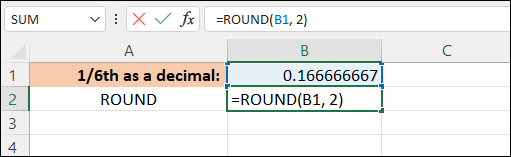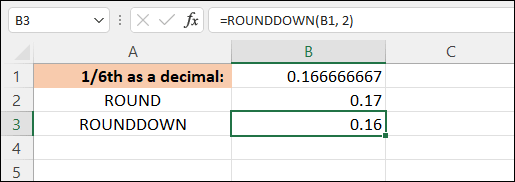Home and Learn: Microsoft Excel Course

# Excel and Rounding Up and Down

Let's look at the various ways you can round up in Excel (and round down).

There are three ROUND functions built in to Excel. They are:

ROUND
ROUNDDOWN
ROUNDUP

(Actually, there's a fourth called MROUND, but we won't worry about that.)

All three need two things between the round brackets of the function: the number you want to round, and how many digits. Let's try them out, one by one.

## ROUND

On a new blank spreadsheet, enter some text in cell A1. Enter the following:

1/6th as a decimal

in cell B1, enter this formula:

=1 / 6

Press enter and you'll get a value of 0.166666667, which is ten numbers:In cell A2, enter the text ROUND.

Click in cell B2 to highlight it. Now click inside the formula bar at the top. Type an = sign, then ROU. You should see a popup appear with all the functions available to you. This one:Select the first one, ROUND. In between the round brackets of the function, enter B1, 2: Your function should be this:

=ROUND(B1, 2)The first value is for your number. Ours was in cell B1. The second number is how many digits we want for rounding purposes, 2 in this case.

Press the enter key on your keyboard and you should see a value of 0.17 appear in cell B2. So Excel has rounded up the 0.166666667 to 0.17 when using the ROUND function.

EXERCISE
Click back into the formula bar, change the 2 to 3 and see what happens. Now change the 3 into a 1 and see what value you get.

## ROUNDDOWN

In cell A3, enter the text ROUNDDOWN. In cell B3, enter this formula:

=ROUNDDOWN(B1, 2)

Just like ROUND, Excel needs you to enter first the number you want to round down followed by how many digits you want.

Press enter and you should get a value of 0.16:## ROUNDUP in Excel

In cell A4, enter the text ROUNDUP. In cell B4, the formula to enter is this:

=ROUNDUP(B1, 2)

Again, we want the contents of cell B1 as our number. And we're rounding up to 2 places.

The value you get when you press enter is 0.17, the same value we got when we used the ROUND function.

The question is, do the two functions (ROUND and ROUNDUP) do the same thing? Do they both round up? The answer is no. ROUND will round down when the value is below 5. For example, enter 0.144 and Excel will round down to 0.14. But enter 0.145 and Excel will round up to 0.15.

## Rounding via the Number panel

The Number panel at the top of Excel can also be used to round a value in a cell up or down. But a note of caution here: When you use the number panel to increase or decrease the number of decimal places, it's just the display that's altered, not the number underneath. For example, click into cell B1. When we divided 1 by 6, we got an answer of 0.166666667. Now, in the Number panel at the top, click Decrease icon:Keep clicking it until the number in B1 reads 0.2.

Now notice the values in your other cells - they don't change! To the other cells, they still get the 0.166666667 and use that for the calculation, and not the 0.2.

In the next lesson below, you'll get some practice with the Time functions you learned about in a previous lesson.

<--Back to the Excel Contents Page

Email us: enquiry at homeandlearn.co.uk